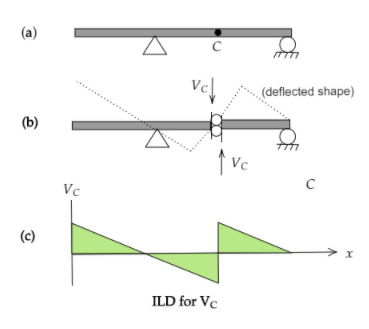top of page
Search
• APSEd

# How to draw Influence Line Diagram (ILD) using Müller-Breslau's principle

First, why ILD? Imagine a moving vehicle on a bridge. For each location, we will get different Shear Force and Bending Moment. To overcome this difficultly, we go for Influence Line Diagram. Müller-Breslau's principle makes it simpler to draw these influence line diagrams.

We can say it in simple terms: ILD is a tool to see the effect of moving loads on a structure.### How is Influence Line Diagram different from Shear Force / Bending Moment Diagrams?

The shear force and bending moment diagram tell us about the SF and BM of the member at any section due to that particular loading case.

But if we talk about the moving live load (like the load case on a bridge or for a range) the location of loading is not the same throughout. So for each location, we will get different Shear Force Diagram (SFD) and Bending Moment Diagram (BMD), which is not possible to draw practically. To overcome this difficulty we draw Influence Line Diagram (ILD).

To understand it better, let’s assume a case.

If there is a bridge deck and the midpoint of the span is C. One vehicle is moving along the bridge. And we need to find out the variation of BM and SF at C as the load passes the member.

This type of question is very easily solved using Influence Line Diagram.The basic difference between SFD/BMD and the ILD is, in SFD/BMD we get the SF and BM across various sections but in case of ILD the section is fixed and the live load is moving.

By using ILD, we can find the section where the SF or BM is maximum due to that particular moving load. We also can find out the magnitude of maximum SF and max BM.

Before jumping on to Müller-Breslau's Principle, you can understand the influence line diagram from basics here.

## Müller-Breslau's Principle

Muller Breslau principle makes it simpler to draw these influence line diagrams.

What is the principle?

The influence line for a function (Reaction, SF, BM, etc.) is to the same scale as the deflected shape of the beam where the beam is acted upon by that function.

Let us see how easy it gets with an example. If you prefer to watch it as a video, you can have a look here: Untold Concept of Influence Line Diagram

### I. Finding ILD for ReactionThe Influence Line Diagram for the function R(A) is the deflected shape of the beam due to R(A) as shown.### II. Finding ILD for shear at C

Next, we will find the ILD for shear at C. First of all, remove the capacity to resist shear at C by providing a slider. After this, we applied shear in a positive sense.### III. Finding ILD for moment at C

If we want to draw the ILD for the moment at C. Same thing we have to follow, remove the capacity at section C, and put a hinge to resist MC. Then we apply the virtual bending in a positive sense, letting it rotate.## Challenge Question on Influence Line Diagram

Try this question